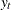The TIMEDATA Procedure

Time Series Differencing

After you optionally transform the series, you can simply or seasonally difference the accumulated series by using the VAR statement DIF= and SDIF= options. For example, supposeis a monthly time series. The following examples of the DIF= and SDIF= options demonstrate how to simply and seasonally difference the time series.

```   dif=(1) sdif=(1)
dif=(1,12)
```

Additionally, whenis strictly positive and the TRANSFORM=, DIF=, and SDIF= options are combined in the VAR statements, the transformation operation is performed before the differencing operations.